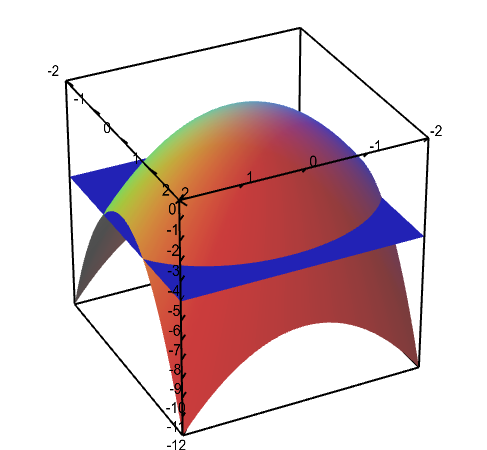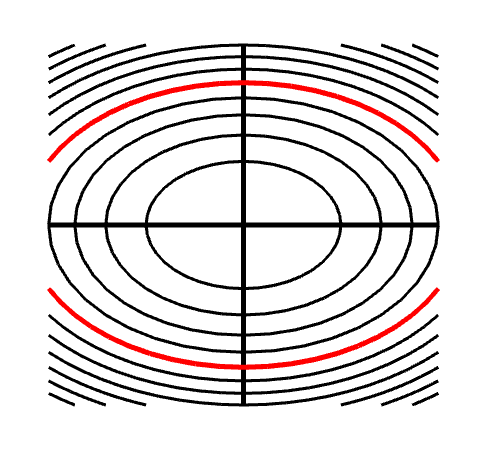# Math Insight

### Applet: Level curves of an elliptic paraboloid shown with graphThe graph of the function $f(x,y)=-x^2-2y^2$ is shown is the first panel along with a level curve plot in the second panel. The level curve $f(x,y)=c$ is shown in red in the level curve plot, which is the same as the slice of the graph $z=f(x,y)$ by the plane $z=c$. You can change $c$ by dragging the plane slicing the graph up or down with the mouse. You can also change $c$ by dragging the red level curve.